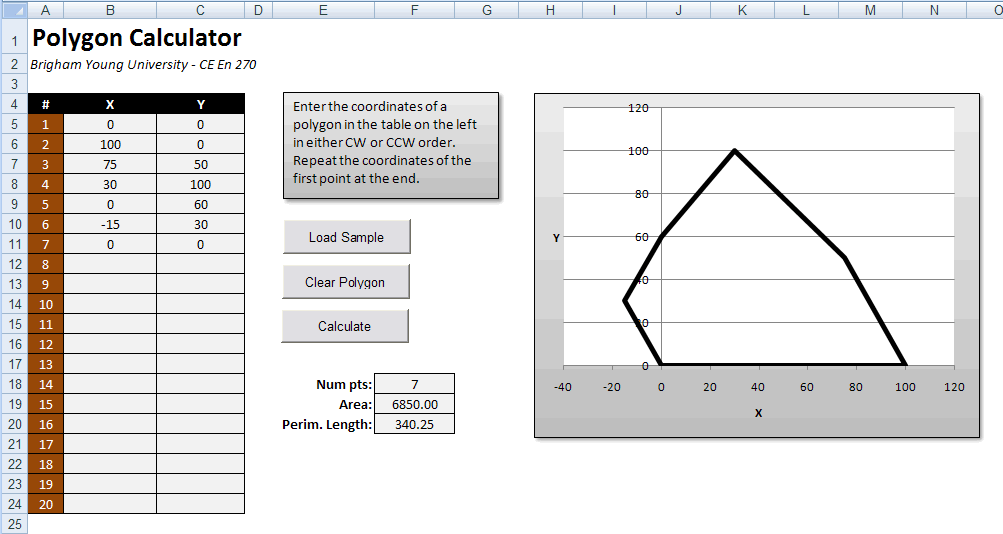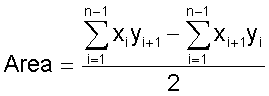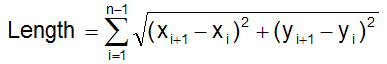# HW#17 - Polygon Calculations

When writing spreadsheets, we often encounter situations where we need to allow the user to enter a list of values where the length of the list may vary from one situation to the next.  We then perform some operation on the list.  This is difficult or impossible in most cases with standard spreadsheet formulas and functions.  However, it is quite simple with VBA code.  This assignment involves writing code that operates on a variable length list.  It also involves using one-dimensional variable arrays.

The following spreadsheet computes the area and perimeter length of an n-sided polygon given a list of x and y coordinates representing the vertices of the polygon:This button should clear the contents of the coordinate table and then load a sample polygon (you can use the values shown above if you wish).  This will be a useful button while you are debugging your code.

2. Clear Polygon Button

This button should simply clear the contents of the coordinate table.

3. Calculate Button

The calculate button should read the coordinates into a pair of x and y arrays.  It should then compute and display the area and perimeter length of the polygon.

To read the arrays, you should first enter the Count function in cell F18 and then read the value of this cell from your VB code to determine the number of items (numpts) in either the x or the y column.  You should then dynamically allocate two arrays x and y to range from 1 to numpts.  Then use a For Loop to load the points into slots 1 to numpts in the arrays.

Computing the Area

The area of the polygon can then be computed as:This equation assumes that the first set of coordinates is repeated at the end of the list as shown in the example above.

The coordinates can be entered in either clockwise or counter-clockwise order.  Depending on the ordering, the area will be either positive or negative.  Just take the absolute value of the result.

Computing the Perimeter Length

To compute the length, just sum the length of the edges making up the polygon as follows:where n is the number of points in the array.  Once again, this equation assumes that the first set of coordinates is repeated at the end.